# College Physics

Physics & Astronomy

## Quiz 4 : Direct-Current CircuitsLooking for Introductory Physics Homework Help?

## Quiz 4 :Direct-Current Circuits

Question TypeIs the direction of current in a battery always from the negative terminal to the positive one Explain.
Free
Essay

No, it depends on the emf of the battery. If the battery has a more emf, than the source emf then the battery acts as a source to the circuit. In such situation, the current will flows from the negative terminal to the positive terminal of the battery. If the battery has less emf than the source emf, then the current will flows from the positive terminal of the battery to the negative terminal of the battery.

TagsA battery having an emf of 9.00 V delivers 117 mA when connected to a 72.0- load. Determine the internal resistance of the battery.
Free
Essay

The relation between the current I and the emf of the battery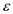in presence of internal resistance r of the battery is shown below.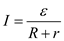Here, R is the load resistance and r is the internal resistance.
Re-arrange the equation to solve for r.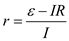Substitute 9.00 V for, 0.117 mA for I , and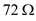.Therefore, the internal resistance r of the battery is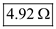.

TagsGiven three lightbulbs and a battery, sketch as many different circuits as you can.
Free
Essay

Three light bulbs are in series connection with a battery supply of V is as shown in figure 1.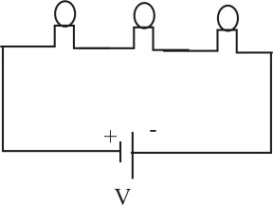Figure 1
Three Light bulbs are in parallel connection with a battery supply of V is as shown in figure 2.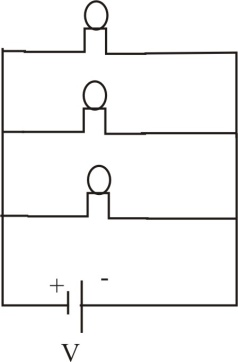Figure 2
Two Light bulbs are in series connection and the resultant brightness of these two in parallel with third light bulb with a battery supply V is as shown in figure 3.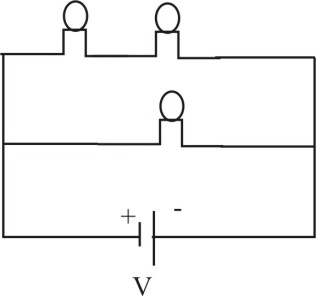Figure 3
Two Light bulbs are in parallel connection and the resultant brightness of these two in series with third light bulb with a battery supply V is as shown in figure 4.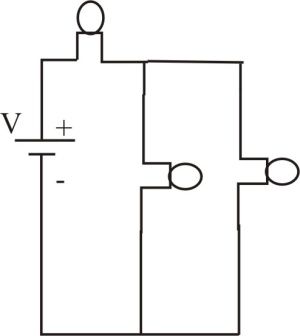Figure 4
Therefore, the possible connections obtained for three bulbs and a battery are 4.

TagsThree 9.0- resistors are connected in series with a 12-V battery. Find (a) the equivalent resistance of the circuit and (b) the current in each resistor. (c) Repeat for the case in which all three resistors are connected in parallel across the battery.
Essay
TagsSuppose the energy transferred to a dead battery during charging is W. The recharged battery is then used until fully discharged again. Is the total energy transferred out of the battery during use also W
Essay
TagsA lightbulb marked "75 W [at] 120 V" is screwed into a socket at one end of a long extension cord in which each of the two conductors has a resistance of 0.800 V. The other end of the extension cord is plugged into a 120-V outlet. (a) Draw a circuit diagram, and (b) find the actual power of the bulb in the circuit described.
Essay
TagsA short circuit is a circuit containing a path of very low resistance in parallel with some other part of the circuit. Discuss the effect of a short circuit on the portion of the circuit it parallels. Use a lamp with a frayed line cord as an example.
Essay
Tags(a) Find the current in an 8.00- resistor connected to a battery that has an internal resistance of 0.15 if the voltage across the battery (the terminal voltage) is 9.00 V. (b) What is the emf of the battery
Essay
TagsConnecting batteries in series increases the emf applied to a circuit. What advantage might there be to connecting them in parallel
Essay
Tags(a) Find the equivalent resistance between points a and b in Figure P18.5. (b) Calculate the current in each resistor if a potential difference of 34.0 V is applied between points a and b.
Essay
TagsIf electrical power is transmitted over long distances, the resistance of the wires becomes significant. Why Which mode of transmission would result in less energy loss, high current and low voltage or low current and high voltage Discuss.
Essay
TagsConsider the combination of resistors shown in Figure P18.6. (a) Find the equivalent resistance between point a and b. (b) If a voltage of 35.0 V is applied between points a and b , find the current in each resistor.
Essay
TagsIf you have your headlights on while you start your car, why do they dim while the car is starting
Essay
TagsTwo resistors connected in series have an equivalent resistance of 690 . When they are connected in parallel, their equivalent resistance is 150 . Find the resistance of each resistor.
Essay
TagsTwo sets of Christmas lights are available. For set A, when one bulb is removed, the remaining bulbs remain illuminated. For set B, when one bulb is removed, the remaining bulbs do not operate. Explain the difference in wiring for the two sets.
Essay
TagsConsider the circuit shown in Figure P18.8. (a) Calculate the equivalent resistance of the 10.0- and 5.00- resistors connected in parallel. (b) Using the result of part (a), calculate the combined resistance of the 10.0- , 5.00- , and 4.00- resistors. (c) Calculate the equivalent resistance of the combined resistance found in part (b) and the parallel 3.00- resistor. (d) Combine the equivalent resistance found in part (c) with the 2.00- resistor. (e) Calculate the total current in the circuit. (f) What is the voltage drop across the 2.00- resistor (g) Subtracting the result of part (f) from the battery voltage, find the voltage across the 3.00-V resistor. (h) Calculate the current in the 3.00-V resistor.
Essay
TagsWhy is it possible for a bird to sit on a high-voltage wire without being electrocuted (See Fig. CQ18.9.)
Essay
TagsConsider the circuit shown in Figure P18.9. Find (a) the potential difference between points a and b and (b) the current in the 20.0- resistor.
Essay